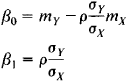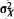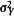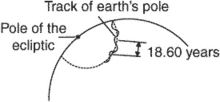# regression

(redirected from regressive)
Also found in: Dictionary, Thesaurus, Medical, Financial.

## regression,

in psychology: see defense mechanismdefense mechanism,
in psychoanalysis, any of a variety of unconscious personality reactions which the ego uses to protect the conscious mind from threatening feelings and perceptions.
.

## Regression

an inherent part of the evolutionary process and a special direction of the process. The level of organization of the living system undergoing regression determines the phenomenon’s evolutionary significance. Biological regression, as defined by A. N. Severtsov, involves a decrease in the number of individuals within a species or other group and a decrease in a particular group’s area of distribution, number of habitats, and number of subgroups. It may culminate in extinction of a group or in its conversion to a rare relict. In nature, the biological regression of groups least capable of competition often results in the flourishing of other groups. For example, previously prevalent sago palms and dinosaurs were superseded by other forms. The palms are preserved in the form of “living fossils,” and the dinosaurs are extinct.

Morphophysiological regression is a process of partial or complete reduction of organs and systems of organs that have lost their adaptive significance. Owing to morphophysiological regression an organism is freed from characters or organs that were useful in the past but have become useless or even harmful under changed conditions of life. There are three directions in morphophysiological regression: (1) lost formations are replaced by new, more efficient ones (for example, replacement of the notochord by a cartilaginous skeleton and later by a bony skeleton in the process of vertebrate evolution); (2) the loss of certain characters entails the development of new ones that are more appropriate to a new environment (replacement of gills by lungs when vertebrates emerged onto dry land; compensatory replacement of eyes by tactile organs in cave fauna); and (3) some structures and functions are lost and not replaced (observed, for example, when environmental conditions are simplified owing to a transition to sessile life or to internal parasitism).

The first two directions of morphophysiological regression, which lead to complexity of organization or to its maintenance on the previous level, are necessary for evolutionary progress. The third direction leads to general simplification of organization, because the regression affects not isolated characters or organs but the entire organization of the individual. This evolutionary direction was called general degeneration by A. N. Severtsov and katamorphosis by I. I. Shmal’gauzen.

### REFERENCES

Severtsov, A. N. Glavnye napravleniia evoliutsionnogo protsessa, 3rd ed. Moscow, 1967.
Shmal’gauzen, I. I. Problemy darvinizma, 2nd ed. Leningrad, 1969.

## Regression

a type of development characterized by a shift from a higher to a lower state. Regression is marked by degeneration, decline in the level of organization, and loss of the ability to perform various essential functions. Regression also includes stagnation and a return to obsolete forms and structures. In its direction, regression is the opposite of progress. But between them there exists a complex, multifaceted link: on the one hand, certain regressive changes may occur within the overall progressive development of a system; and on the other hand, while regressive changes are increasing within a system as a whole, certain elements of the system may maintain a progressive direction of development.

In the development of society, the possibility of regression is inherent in the contradictory nature of the historical process itself. V. I. Lenin emphasized that “history is moving in zigzags and by roundabout ways” (Poln. sobr. soch., 5th ed., vol. 36, p. 82). Reactionary classes and forces may temporarily prevail over progressive forces, as in periods of reaction or the growth of fascism. However, these regressive phenomena are merely a product of the dissolution of obsolete social forms: new forms that have absorbed all that was enduring and valuable in their predecessors have already appeared to replace them. The dissolution of a given phenomenon does not interrupt the developmental process of the more general system; it is even one of the essential preconditions of the developmental process.

### REFERENCES

See references under progress.

I. S. KON and L. SEREBRIAKOV

## Regression

in probability theory and mathematical statistics, the dependence of the mean value of some variable on one or more other variables. Unlike the purely functional relation y = f(x), where to each value of the independent variable x there corresponds a particular value of the variable y, in a regression relation different values of y may correspond to a single value of x, depending on the case. If ni values

yil, …, yini

of the variable y are observed for each value of x = xi, the dependence of the arithmetic meanson xi is a regression in the statistical sense of the term. An example of this type of dependence is the relationship between the mean diameter and height of pine trees as illustrated in Table 1 of the article CORRELATION.

In probability theory, the basis for the study of regressions is the probabilistic nature of the dependence that connects two random variables X and Y having a joint probability distribution: for every fixed value of X = x, the variable Y is a random variable with a specific conditional probability distribution that depends on the value of x. The regression of Y on X is determined by the conditional mathematical expectation of Y, calculated under the condition that X = x:

E (Y\x) = u(x)

The equation y = u(x), in which x plays the role of an “independent” variable, is called a regression equation, and the corresponding curve is called the regression line of Y on X. The accuracy with which the regression equation of y on X reflects the mean variation of Y as X varies is measured by the conditional variance of Y, calculated for each value of X = x:

D(y\x) = σ2(x)

If σ2(x) = 0 for all values of x, it can be confidently asserted that y and X are connected by the strict functional relation Y = u(X). If σ2(x) ≠ 0 for all values of x and if u(x) does not depend on x, we say that there is no regression of Y on X. The regression of X on Y and, in particular, the regression equation x = v(y), where v(y) = E(X ǀ Y = y), are defined in an analogous manner. The functions y = u(x) and x = v(y) are not, in general, inverses of each other.

Regression lines have a remarkable property: of all the real functions f(x), the function f(x) = u(x) has the smallest mathematical expectation E [Y-f(X)]2. This means that the regression of Y on X yields the best representation, in the above sense, of the variable Y with respect to the variable X. This property is used to predict Y on the basis of X; that is, if the value of Y cannot be directly observed and the experiment permits only the component X of the vector (X, Y) to be observed, then u(X) is used as the predicted value of Y.

In the simplest case, the regression of Y on X is linear:

E(Y\x) = β0 + β1x

The coefficients β0 and β1, called the regression coefficients, are determined from the equationswhere mx and mY are the mathematical expectations of X and y, respectively,andare the variances of X and Y, respectively, and ρ is the correlation coefficient of X and Y. The regression equation here is given by the formulaWhen the joint distribution of X and Y is normal, both regression lines y = u(x) and x = v(y) are straight lines.

If the regression of Y on X is not linear, the last equation becomes a linear approximation of the true regression equation: the mathematical expectation E[y - b0 - b1X]2 attains its minimum with respect to b0 and b1 when b0 = β0 and b1 = β1]. The case of a regression equation that is expressed by a linear combination of particular given functions is encountered especially often:

y = u(x) = β0Φ0 (x) + β1Φ1(x) + … + βm Φm(x)

The parabolic, or polynomial, regression—in which Φ0(x) = 1, Φ1(x) = x,…, Φm(x) = xm —is of particular importance.

The concept of regression is applicable not only to random variables but also to random vectors. In particular, if Y is a random variable and X = (X1, …, Xk) is a random vector with joint probability distribution, the regression of Y on X is determined by the equation

y = u(x1,…,xk)

where

u(x1,…,xk) = E{Y\X1 = x1,,…,Xk = xk}

If

u(x1…,xk) =β0 + β1x1 + … + βkxk

the regression is said to be linear. This form of the regression equation encompasses many types of regressions with a single independent variable; in particular, the polynomial regression of Y on X of degree k reduces to a linear regression of Y on X1, …. Xk if we set Xk = Xk.

A simple example of a regression of Y on X is the relation between Y and X expressed by Y = u(X) + δ, where u(x) = E(YI X = x) and the random variables X and δ are independent. This representation is useful in designing an experiment to study the functional relation y = u(x) between two nonrandom variables y and x.

In practice, the regression coefficients in the equation y= u(x) are usually unknown and are estimated from experimental data.

The term “regression” was first used in a special sense by the English statistician F. Galton in 1886 in his theory of inheritance. He applied the phrase “regression to mediocrity” to the relationship between the heights of tall parents and the heights of the children of such parents: the children of parents whose heights exceed the mean height of all parents by a units have on the average a height that exceeds the mean height of all children by less than a units.

### REFERENCES

Cramer, H. Matematicheskie metody statistiki. Moscow, 1948. (Translated from English.)
Kendall, M. G., and A. Stuart. Statisticheskie vyvody i sviazi. Moscow, 1973. (Translated from English.)

A. V. PROKHOROV

## Regression

the retreat of the sea from its coasts. Regression occurs as a result of an uplifting of the land, a subsidence of the ocean floor, or a decrease in the water volume of ocean basins, which happens, for example, during glacial epochs. Throughout the history of the earth there have been numerous regressions in many different regions.

## regression

[ri′gresh·ən]
(geology)
The theory that some rivers have sources on the rainier sides of mountain ranges and gradually erode backward until the ranges are cut through.
(oceanography)
Retreat of the sea from land areas, and the consequent evidence of such withdrawal.
(psychology)
A mental state and a mode of adjustment to difficult and unpleasant situations, characterized by behavior of a type that had been satisfying and appropriate at an earlier stage of development but which no longer befits the age and social status of the individual.
(statistics)
Given two stochastically dependent random variables, regression functions measure the mean expectation of one relative to the other.

## regressionThe precession of nodes (e.g., the moon completes a revolution in 18.6 years).

## regression

1. Psychol the adoption by an adult or adolescent of behaviour more appropriate to a child, esp as a defence mechanism to avoid anxiety
2. Astronomy the slow movement around the ecliptic of the two points at which the moon's orbit intersects the ecliptic. One complete revolution occurs about every 19 years
3. Geology the retreat of the sea from the land

## regression

(mathematics)
A mathematical method where an empirical function is derived from a set of experimental data.

## regression

(2)
References in periodicals archive ?
'We will then file a bill that aims to truly reduce the tax burden on the poor majority by repealing the regressive provisions of TRAIN Law...
Prakash also welcomed the apex court's verdict of striking down a 'regressive practice'.
T-R sequence uses the subaerial unconformity as the unconformable portion of the boundary on the basin margin and the maximum regressive surface (MRS) as the correlative conformity farther seaward (Embry and Johannesen, 1992).
As the Government enters tense negotiations on the Budget, Neri said the water charges proposals were "regressive".
Tejas Sharma: "Dear Mallika Sherawat, Please don't come back to a regressive country.
The weakest criticism of HB 2870 is that it's regressive: Smoking rates are highest among the poor, so tobacco taxes hit low-income people the hardest.
In its analysis of Mr Osborne's spending review, the IFS said the changes would reinforce the "regressive" nature of the Government's plans to tackle the deficit, including the pounds 7bn of welfare cuts.
Mr Clegg would like the Budget to be regarded as progressive, rather than regressive as the institute labelled it.
From cuts in public services, to tax breaks for the well-off, the agenda being pushed through this conference is boldly, unapologetically regressive.
Someone needs to dramatize how unfair and regressive this tax is, falling heaviest on working people.
The regressive nature of lotteries is borne out not only by common-sense observations, but by numerous studies and reports.

Site: Follow: Share:
Open / Close